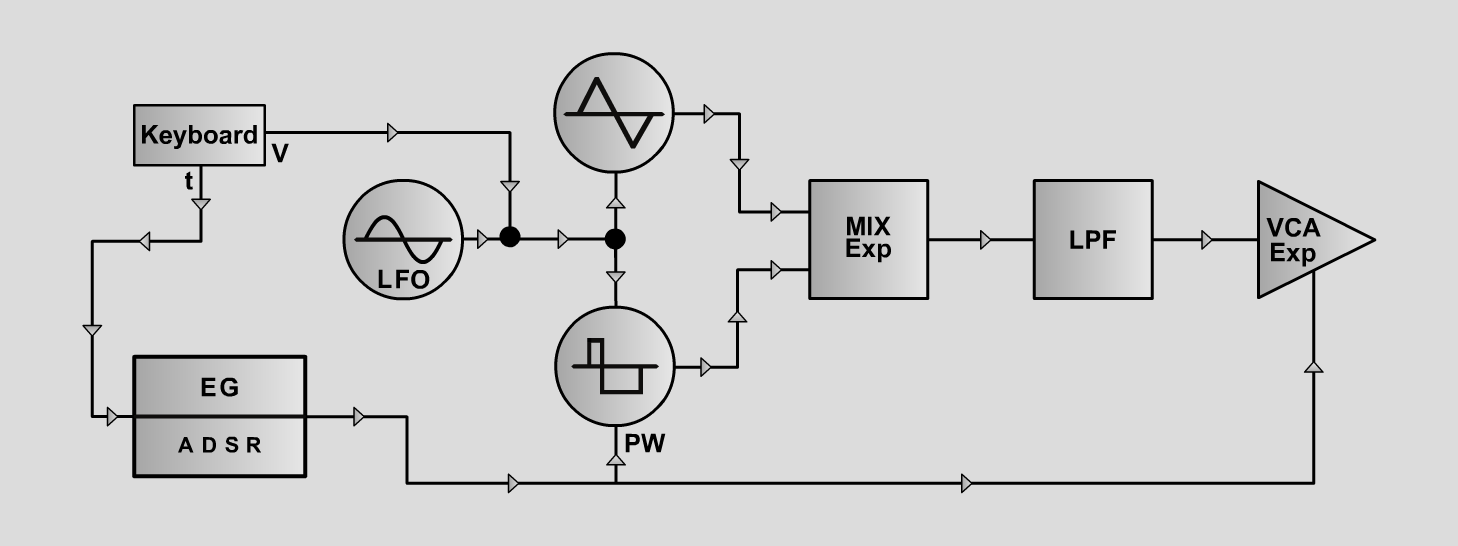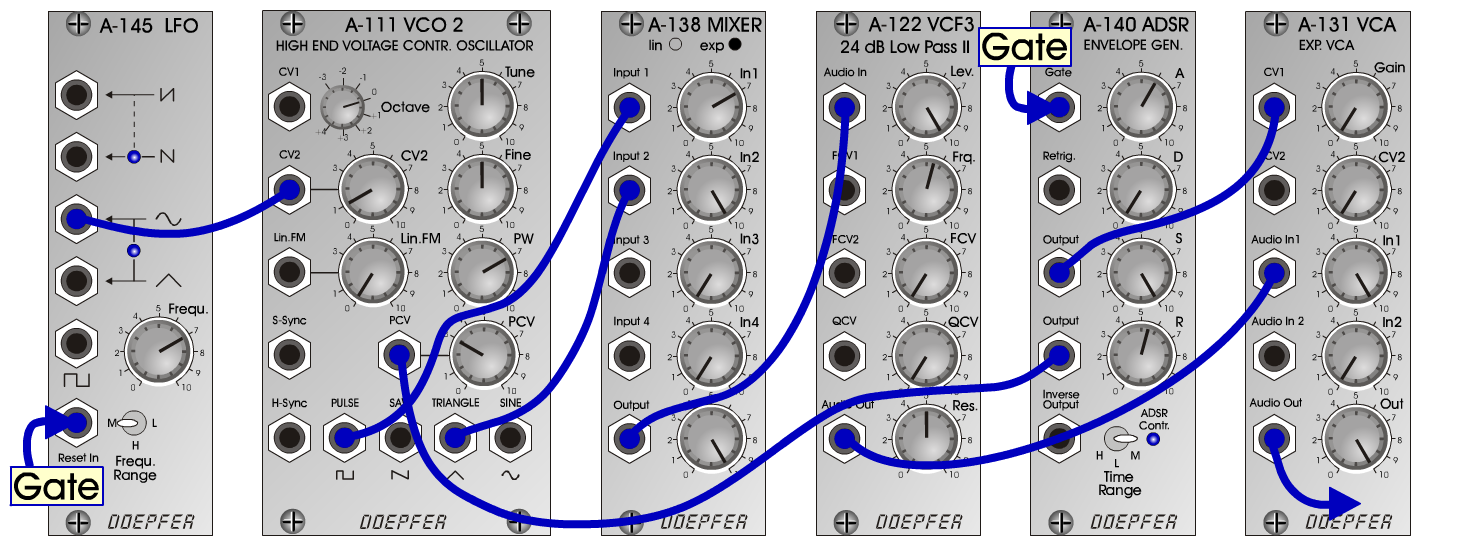D o e p f e r A - 100 Connections: Settings: A-111 (Pulse) <=> A-138exp (Input 1)  A-111 (Triangle) <=> A-138exp (Input 2)  A-138 (Output) <=> A-122 (Audio In)  A-122 (Audio Out) <=> A-131 (Audio In 1)  A-140 (Output 1) <=> A-131 (CV1)  A-140 (Output 2) <=> A-111 (PCV)  A-145 (Sine) <=> A-111 (CV2) A-111 (CV 2 = 1, PW = 7, PCV = 3)  A-138exp (In 1 = 7, In 2 = 10, Out = 10)  A-122 (Level = 10, Freq = 5.5, Res = 5)  A-140 (A = 6, D = 0, S = 10, R = 5.5, Range = M)  A-145 (Frq = 7, Range = M)  A-131 (Gain = 0, Audio In 1 = 10, Audio Out = 10) VocalistSimple: A-111 (CV 2 = 0) Josef MuellerSound samples Bach: Double Violin Concerto (BWV1043) Vocalist (262 Hz) Vocalist (523 Hz) Vocalist (1046 Hz) Vocalist Simple (262 Hz) Vocalist Simple (523 Hz) Vocalist Simple (1046 Hz)